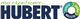# Remove new row during cell validation

GridViewTop achievementsRank 1Veteran
Gone2TheDogs asked on 23 Oct 2017, 08:20 PM

I need to be able to programmatically remove a new row that is still in process (cell validating), so the grid is displayed without the new row.

example:  Grid displays existing rows. User clicks the "add new row" option. Enters an order number in the first cell and either tabs to the next cell or Clicks on a cell on the same row. Cell Validation is called and in the process the order they want to view process is "on hold". In this case, I pop a message and then return to the grid with no changes. The new row should be removed.

I am currently just using e.cancel and putting the user back on the order number field, but this is not ideal. It really should just remove the new row.

Is this possible?

## 3 Answers, 1 is accepted

0Dimitar
Telerik teamanswered on 24 Oct 2017, 09:21 AM
Hi Bob,

You can use the CancelAddNewRow method. Here is an example:
`private ``void ``RadGridView1_CellValidating(``object ``sender, Telerik.WinControls.UI.CellValidatingEventArgs e)`
`{`
`    ``if ``(e.Row ``is ``GridViewNewRowInfo && e.ColumnIndex == 0)`
`    ``{`
`        ``RadMessageBox.Show(``"Hold"``);`
`        ``var newRow = e.Row ``as ``GridViewNewRowInfo;`
`        ``newRow.CancelAddNewRow();`

`    ``}`
`}`

Regards,
Dimitar
Progress Telerik
Try our brand new, jQuery-free Angular components built from ground-up which deliver the business app essential building blocks - a grid component, data visualization (charts) and form elements.
0Gone2TheDogs
Top achievementsRank 1Veteran
answered on 24 Oct 2017, 02:27 PM

That works in that it does remove the new row. However, when I click on "Add New Row" after removing a row, it still has the previous cell value as the current e.value. So, instead of setting the first cell as the edit cell, it just throws the popup message, "on hold" and I'm not able to add a new row.

Unfortunately, I can't display all my code out here as it is owned by the company I work for.

Here is a partial from the CellValidating event:

`01.``'---- NEW ROW -------------`
`02.``If` `TypeOf` `e.Row ``Is` `GridViewNewRowInfo ``Then`
`03.`
`04.``    ``dgvDirects.CurrentRow = dgvDirects.MasterView.TableAddNewRow`
`05.`
`06.`
`07.``    ``If` `IsNothing(e.Value) ``OrElse` `e.Value.ToString.Trim = ``""` `Then`
`08.``        ``dgvDirects.CancelEdit()`
`09.``        ``dgvDirects.currentrow.Cells(``"colDirects_OrderNum"``).IsSelected = ``True`
`10.``        ``Exit` `Sub`
`11.``    ``End` `If`
`12.`
`13.``    ``If` `e.Column.Name = ``"colDirects_OrderNum"` `Then`
`14.`
`15.``        ``'Is this a valid order number?`
`16.``        ``Try`
`17.`
`18.``        ``Catch` `ex ``As` `Exception`
`19.`
`20.``        ``End` `Try`
`21.`
`22.``        ``'Look for Dups on grid`
`23.``        ``Try`
`24.``            ``For` `x ``As` `Integer` `= 0 ``To` `dgvDirects.Rows.Count - 1`
`25.``                ``If` `e.Value = dgvDirects.Rows(x).Cells(``"colDirects_OrderNum"``).Value ``Then`
`26.``                    ``nDupCount += 1`
`27.``                    ``If` `nDupCount >= 1 ``Then`
`28.``                        ``HubertMessageBox.Show(``"Duplicate Order Number found on the grid at row "` `& x + 1, ``Me``.Text, MessageBoxButtons.OK, MessageBoxIcon.Exclamation)`
`29.``                        ``dgvDirects.Rows(x).Cells(``"colDirects_OrderNum"``).IsSelected = ``True`
`30.``                       ``dgvDirects.Rows(x).Cells(``"colDirects_OrderNum"``).Value = ``""`
`31.``                        ``e.cancel = true`
`32.``                        ``'dim newRow = trycast(e.row, gridviewnewrowinfo)`
`33.``                        ``'newRow.CancelAddNewRow()`
`34.``                        ``Exit` `Sub`
`35.``                    ``End` `If`
`36.``                ``End` `If`
`37.``            ``Next`
`38.``        ``Catch` `ex ``As` `Exception`
`39.``        ``End` `Try`
`40.`
`41.``        ``If` `IsNothing(e.Value) ``OrElse` `e.Value.trim = ``""` `Then` `Exit` `Sub`
`42.`
`43.``        ``'If IsNothing(dgvDirects.CurrentRow.Cells("colDirects_OrderNum").Value) OrElse dgvDirects.CurrentRow.Cells("colDirects_OrderNum").Value.trim = "" Then Exit Sub`
`44.`
`45.``        ``Dim` `OrderNum ``As` `String` `= e.Value`
`46.``        ``Try`
`47.``            ``HubertAccount.CallProg(``"MV.ADD.DSORDER"``, OrderNum, miscInOut, errCode, errOut)`
`48.``            ``AddEvent(``"> MV.ADD.DSORDER  OrderNum: "` `& OrderNum)`
`49.``            ``If` `errOut <> ``""` `Then`
`50.``                ``If` `HandleErrorCode(errCode, errOut) = ``False` `Then`
`51.``                    ``e.Cancel = ``True`
`52.``                    ``Exit` `Sub`

And then the code that attempts to load the grid, but returns the popup message, and a flag is returned to cancel the row

`53.``ElseIf` `Not` `IsNothing(Field(miscInOut, DataBASIC.VM, 1)) ``AndAlso` `Field(miscInOut, DataBASIC.VM, 1).Trim.ToLower = ``"cancel"` `Then`
`54.``                            `
`55.``    ``'dim newRow = trycast(e.row, gridviewnewrowinfo)`
`56.``    ``'newRow.CancelAddNewRow()`
`57.`
`58.``    ``e.cancel = true`
`59.``    ``Exit` `Sub`

I hope the information I'm sending is understandable. Let me know if you need screenshots or if I should just open a ticket.

Thanks!

0Dimitar
Telerik teamanswered on 25 Oct 2017, 07:51 AM
Hi Bob,

Could you try moving the code in the CellValidated event handler? On my side, this clears the previous value. If this does not work for you please open a support ticket and attach your project.

I hope this will be useful.

Regards,
Dimitar
Progress Telerik
Try our brand new, jQuery-free Angular components built from ground-up which deliver the business app essential building blocks - a grid component, data visualization (charts) and form elements.
Tags
GridViewGone2TheDogs
Top achievementsRank 1VeteranRank 1Veteran